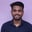Related Tags

golang
function
communitycreator

# What are variadic functions in Go?Parmeshwar Patidar

A variadic function accepts a varying number of arguments.

The ordinary function takes a fixed number of arguments, but we often need a variadic function.

For example, fmt.Println is a variadic function, so it can accept any number of arguments.

## How to define a variadic function

We can define a variadic function by using an ellipsis ( . . .), like . . . T in front of the last parameter in the function signature.

func (someType ...T)


### Example #1

We want a function, sum1, that computes the average of an arbitrary number of integers.

We can use the following signature for this function:

func (nums ...int) int


Take a look below for the full implementation of function sum1, below.

package main

import "fmt"

func sum1(nums ...int) int {
total := 0
for _, num := range nums {
total += num
}
}

func main() {
fmt.Println(sum1())      // with zero (empty) argument
fmt.Println(sum1(1, 2)) // more than zero argument
}

a variadic function which takes zero or more arguments

### Example #2

Now, our requirement is slightly changed. We want a function sum2 that takes at least one integer and calculates the sum.

To solve this, we can use the first argument as a fixed argument, followed by a variadic parameter.

package main

import "fmt"

func sum2(num1 int, nums ...int) int {
total := num1
for _, num := range nums {
total += num
}
}

func main() {
fmt.Println(sum2())     // gives error

fmt.Println(sum2(0))
fmt.Println(sum2(1, 2, 3, 4, 5))
}

a variadic function which takes at least zero arguments

Try commenting line 14 to successfully run the program above.

### How to pass the slice type to a variadic function

We can also pass slice S []T as an argument to . . . T, using syntax S . . . to unpack slice elements.

Suppose we have a slice S of integer:

S := []int{1,2,3,4,5}


We can pass slice S to sum1 and sum2, as shown:

package main

import "fmt"

func sum1(nums ...int) int {
total := 0
for _, num := range nums {
total += num
}
}

func sum2(num1 int, nums ...int) int {
total := num1
for _, num := range nums {
total += num
}
}

func main() {
S := []int{1,2,3,4,5}
fmt.Println(sum1(S...))

// first argument should be normal
fmt.Println(sum2(0, S...))
}

Pass slice as an argument to variadic function

## Conclusion

As we have seen, a variadic function is an inevitable feature of any programming language as it provides versatility.

RELATED TAGS

golang
function
communitycreator

CONTRIBUTORParmeshwar Patidar
RELATED COURSES

View all Courses

Keep Exploring

Learn in-demand tech skills in half the time